Plotting methods for an object of class 'mclustDensity'. Available graphs are plot of BIC values and density for univariate and bivariate data. For higher data dimensionality a scatterplot matrix of pairwise densities is drawn.

# S3 method for densityMclust
plot(x, data = NULL, what = c("BIC", "density", "diagnostic"), ...)

plotDensityMclust1(x, data = NULL, col = gray(0.3), hist.col = "lightgrey",
hist.border = "white",  breaks = "Sturges", ...)

plotDensityMclust2(x, data = NULL, nlevels = 11, levels = NULL,
prob = c(0.25, 0.5, 0.75),
points.pch = 1, points.col = 1, points.cex = 0.8, ...)

plotDensityMclustd(x, data = NULL, nlevels = 11, levels = NULL,
prob = c(0.25, 0.5, 0.75),
points.pch = 1, points.col = 1, points.cex = 0.8,
gap = 0.2, ...)

Arguments

x

An object of class 'mclustDensity' obtained from a call to densityMclust function.

data

Optional data points.

what

The type of graph requested:

"density" =

a plot of estimated density; if data is also provided the density is plotted over data points (see Details section).

"BIC" =

a plot of BIC values for the estimated models versus the number of components.

"diagnostic" =

diagnostic plots (only available for the one-dimensional case, see densityMclust.diagnostic)

col

The color to be used to draw the density line in 1-dimension or contours in higher dimensions.

hist.col

The color to be used to fill the bars of the histogram.

hist.border

The color of the border around the bars of the histogram.

breaks

See the argument in function hist.

points.pch, points.col, points.cex

The character symbols, colors, and magnification to be used for plotting data points.

nlevels

An integer, the number of levels to be used in plotting contour densities.

levels

A vector of density levels at which to draw the contour lines.

prob

A vector of probability levels for computing HDR. Only used if type = "hdr" and supersede previous nlevels and levels arguments.

gap

Distance between subplots, in margin lines, for the matrix of pairwise scatterplots.

...

Details

The function plot.densityMclust allows to obtain the plot of estimated density or the graph of BIC values for evaluated models.

If what = "density" the produced plot dependes on the dimensionality of the data.

For one-dimensional data a call with no data provided produces a plot of the estimated density over a sensible range of values. If data is provided the density is over-plotted on a histogram for the observed data.

For two-dimensional data further arguments available are those accepted by the surfacePlot function. In particular, the density can be represented through "contour", "hdr", "image", and "persp" type of graph. For type = "hdr" Highest Density Regions (HDRs) are plotted for probability levels prob. See hdrlevels for details.

For higher dimensionality a scatterplot matrix of pairwise projected densities is drawn.

Luca Scrucca

Examples

# \donttest{
dens <- densityMclust(faithful$waiting)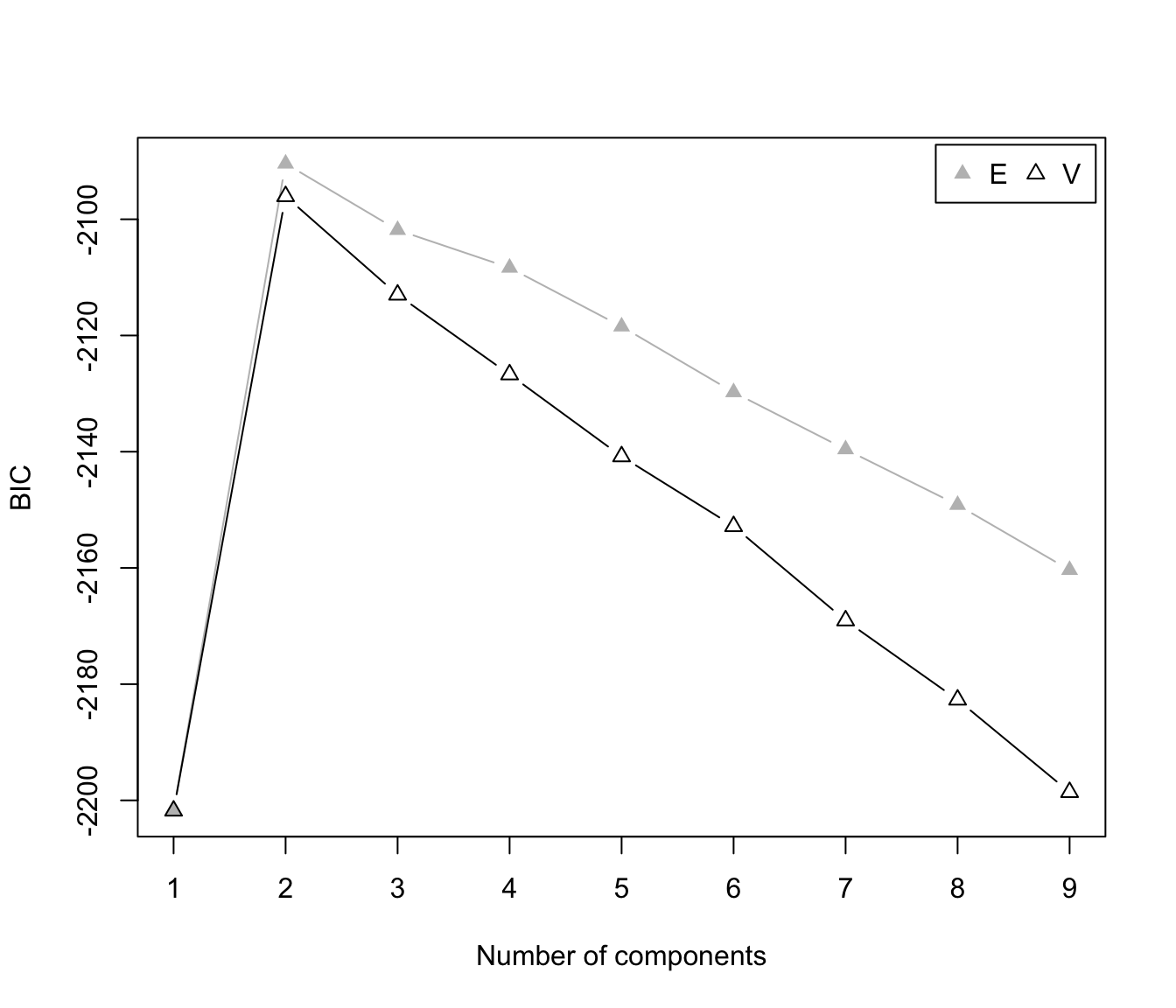summary(dens) #> ------------------------------------------------------- #> Density estimation via Gaussian finite mixture modeling #> ------------------------------------------------------- #> #> Mclust E (univariate, equal variance) model with 2 components: #> #> log-likelihood n df BIC ICL #> -1034.002 272 4 -2090.427 -2099.576 summary(dens, parameters = TRUE) #> ------------------------------------------------------- #> Density estimation via Gaussian finite mixture modeling #> ------------------------------------------------------- #> #> Mclust E (univariate, equal variance) model with 2 components: #> #> log-likelihood n df BIC ICL #> -1034.002 272 4 -2090.427 -2099.576 #> #> Mixing probabilities: #> 1 2 #> 0.3609461 0.6390539 #> #> Means: #> 1 2 #> 54.61675 80.09239 #> #> Variances: #> 1 2 #> 34.44093 34.44093 plot(dens, what = "BIC", legendArgs = list(x = "topright"))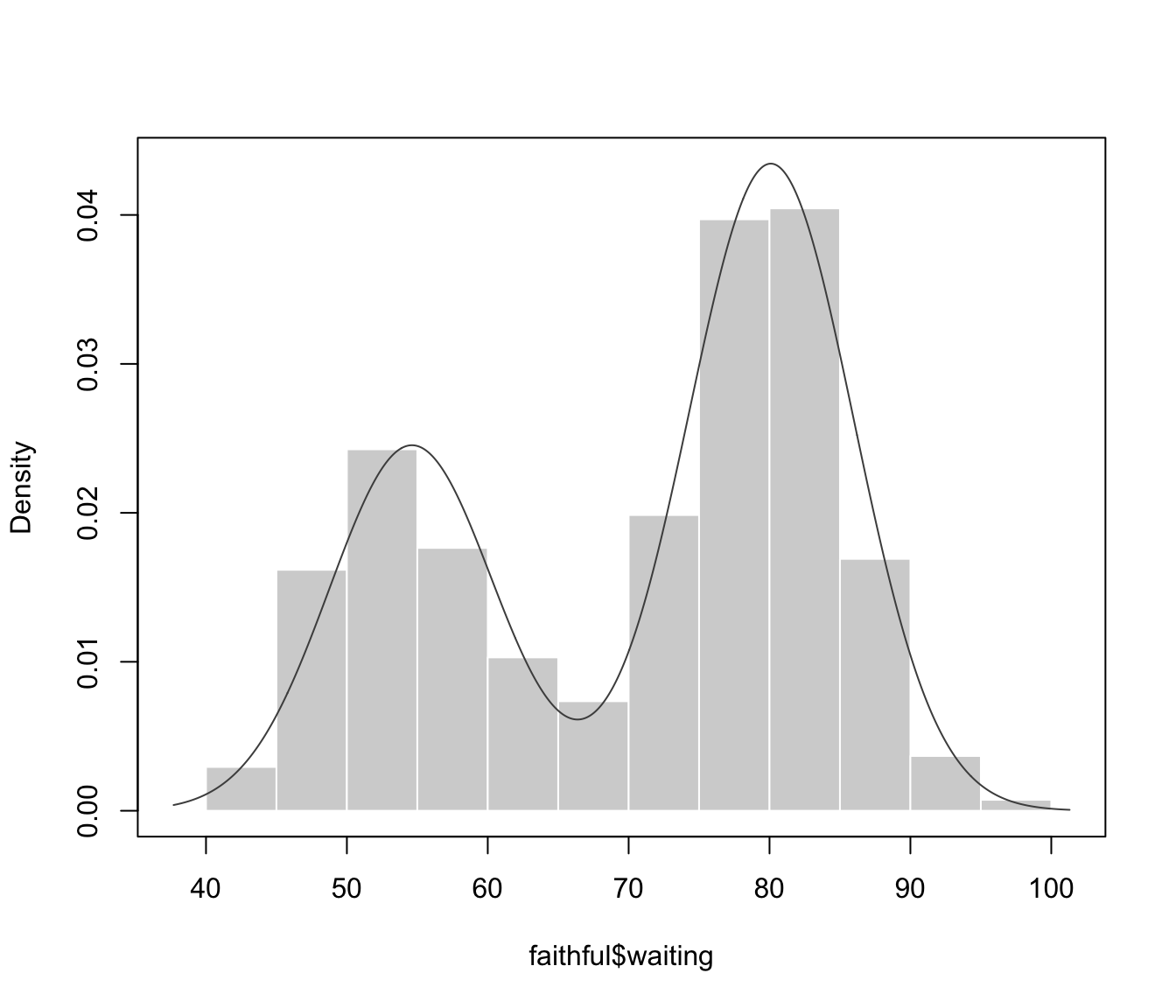plot(dens, what = "density", data = faithful$waiting)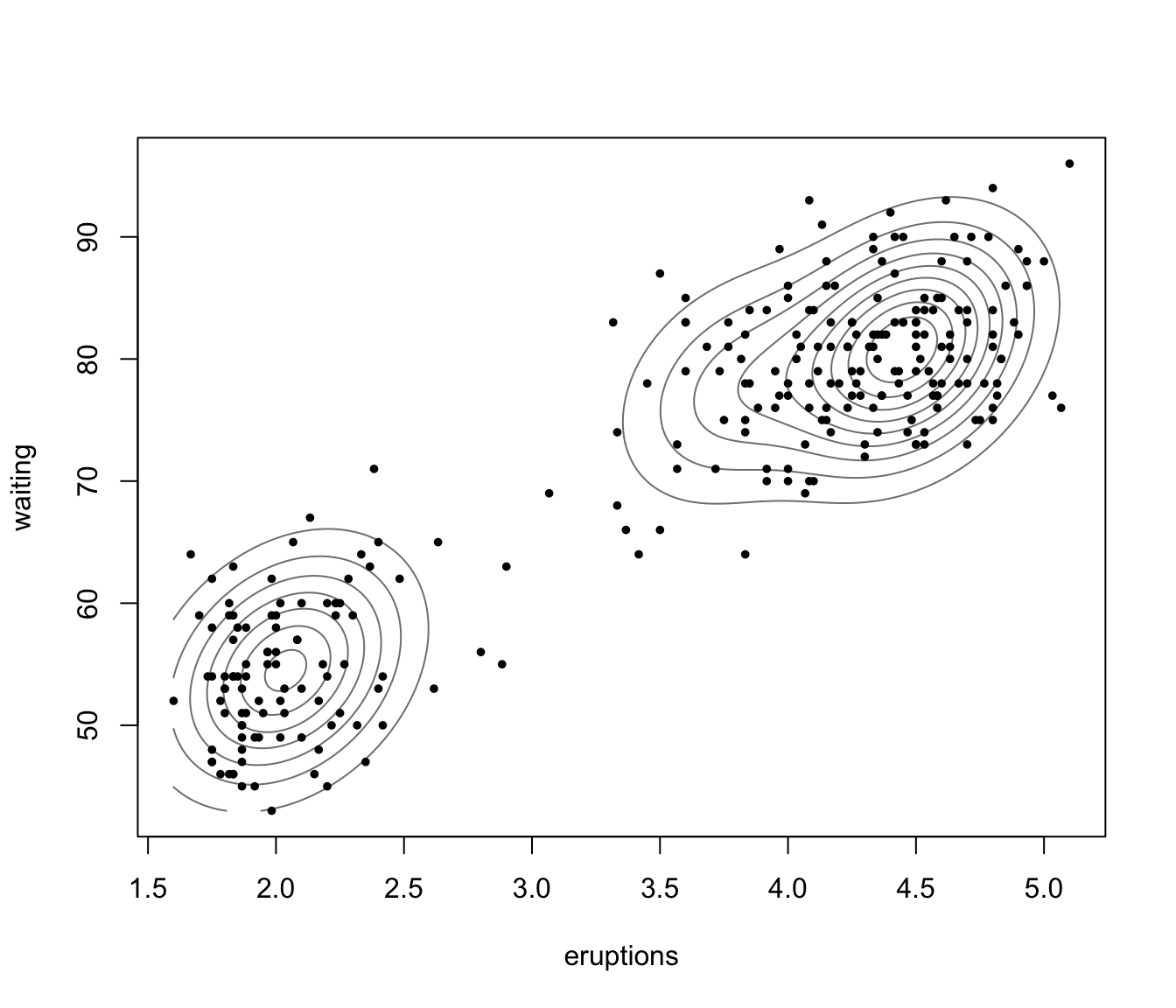dens <- densityMclust(faithful)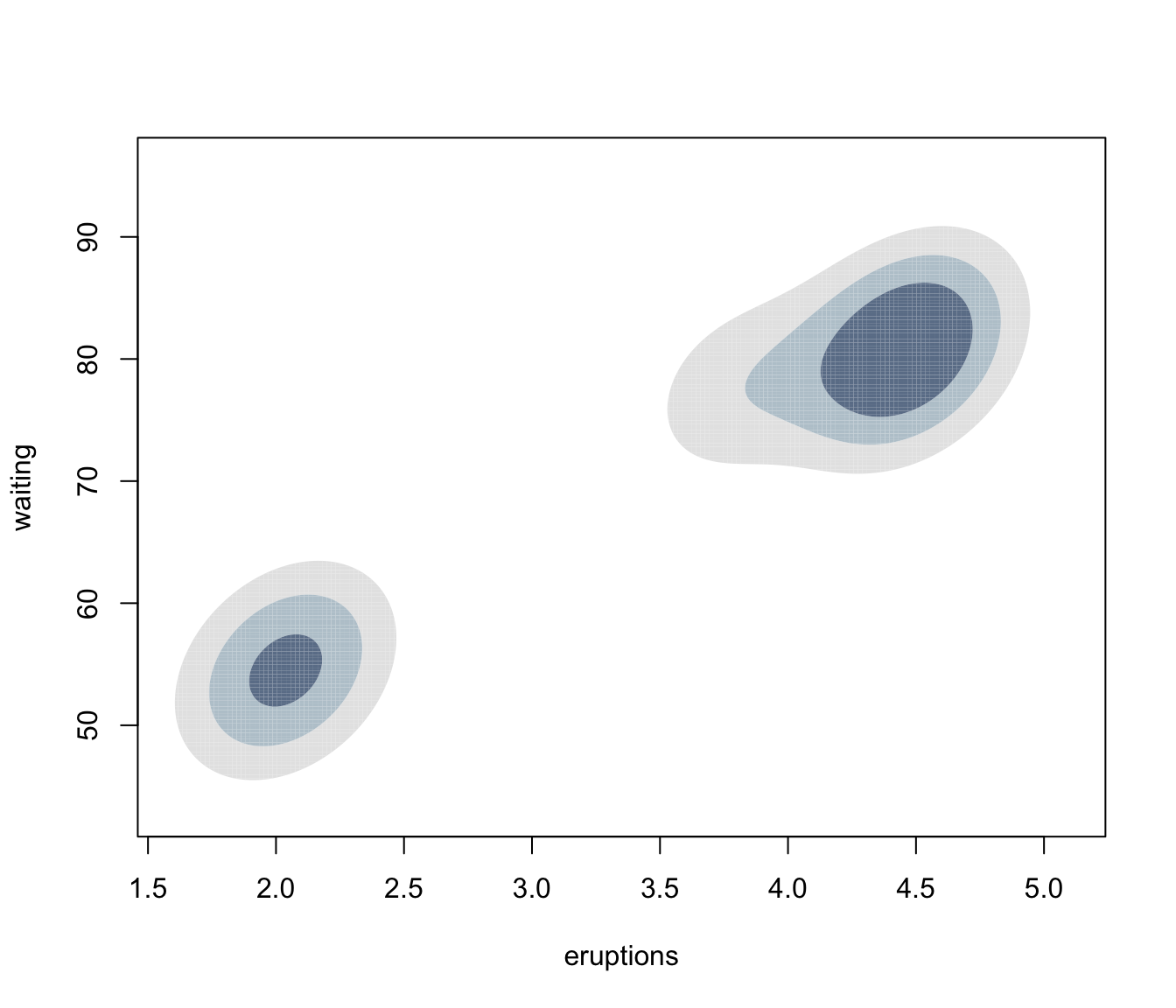summary(dens)
#> -------------------------------------------------------
#> Density estimation via Gaussian finite mixture modeling
#> -------------------------------------------------------
#>
#> Mclust EEE (ellipsoidal, equal volume, shape and orientation) model with 3
#> components:
#>
#>  log-likelihood   n df       BIC       ICL
#>       -1126.326 272 11 -2314.316 -2357.824
summary(dens, parameters = TRUE)
#> -------------------------------------------------------
#> Density estimation via Gaussian finite mixture modeling
#> -------------------------------------------------------
#>
#> Mclust EEE (ellipsoidal, equal volume, shape and orientation) model with 3
#> components:
#>
#>  log-likelihood   n df       BIC       ICL
#>       -1126.326 272 11 -2314.316 -2357.824
#>
#> Mixing probabilities:
#>         1         2         3
#> 0.1656784 0.3563696 0.4779520
#>
#> Means:
#>                [,1]      [,2]      [,3]
#> eruptions  3.793066  2.037596  4.463245
#> waiting   77.521051 54.491158 80.833439
#>
#> Variances:
#> [,,1]
#>            eruptions    waiting
#> eruptions 0.07825448  0.4801979
#> waiting   0.48019785 33.7671464
#> [,,2]
#>            eruptions    waiting
#> eruptions 0.07825448  0.4801979
#> waiting   0.48019785 33.7671464
#> [,,3]
#>            eruptions    waiting
#> eruptions 0.07825448  0.4801979
#> waiting   0.48019785 33.7671464
plot(dens, what = "density", data = faithful,
drawlabels = FALSE, points.pch = 20)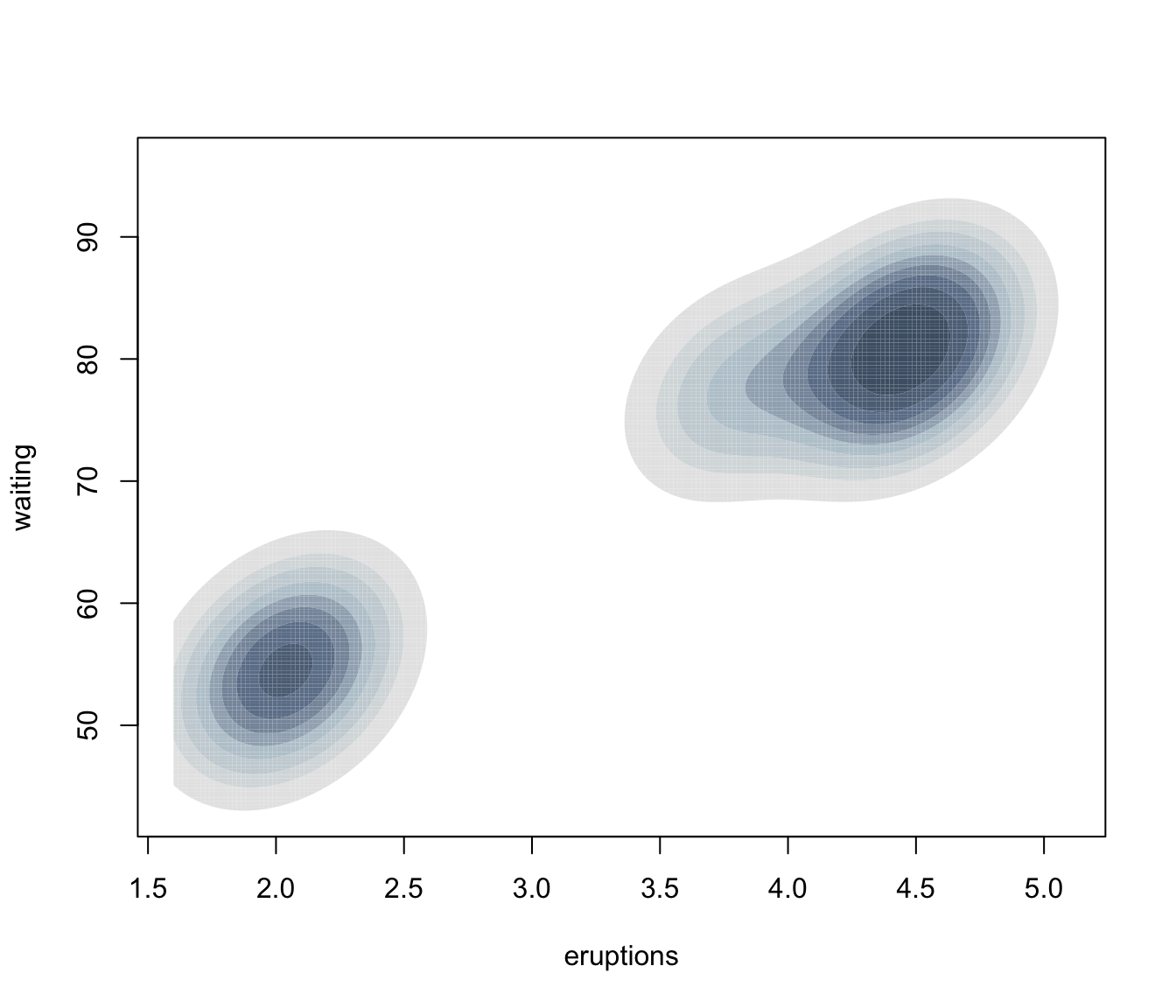plot(dens, what = "density", type = "hdr")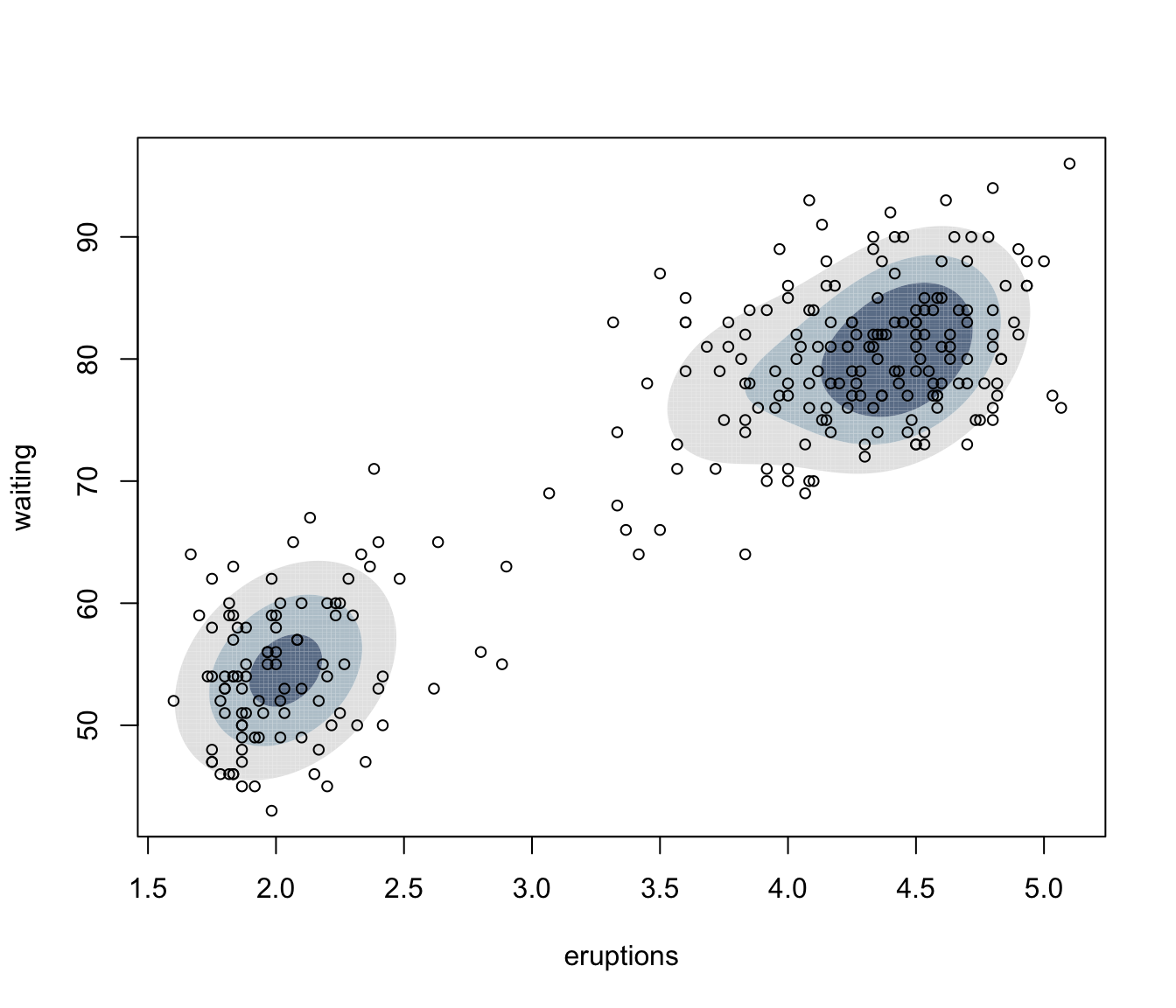plot(dens, what = "density", type = "hdr", prob = seq(0.1, 0.9, by = 0.1))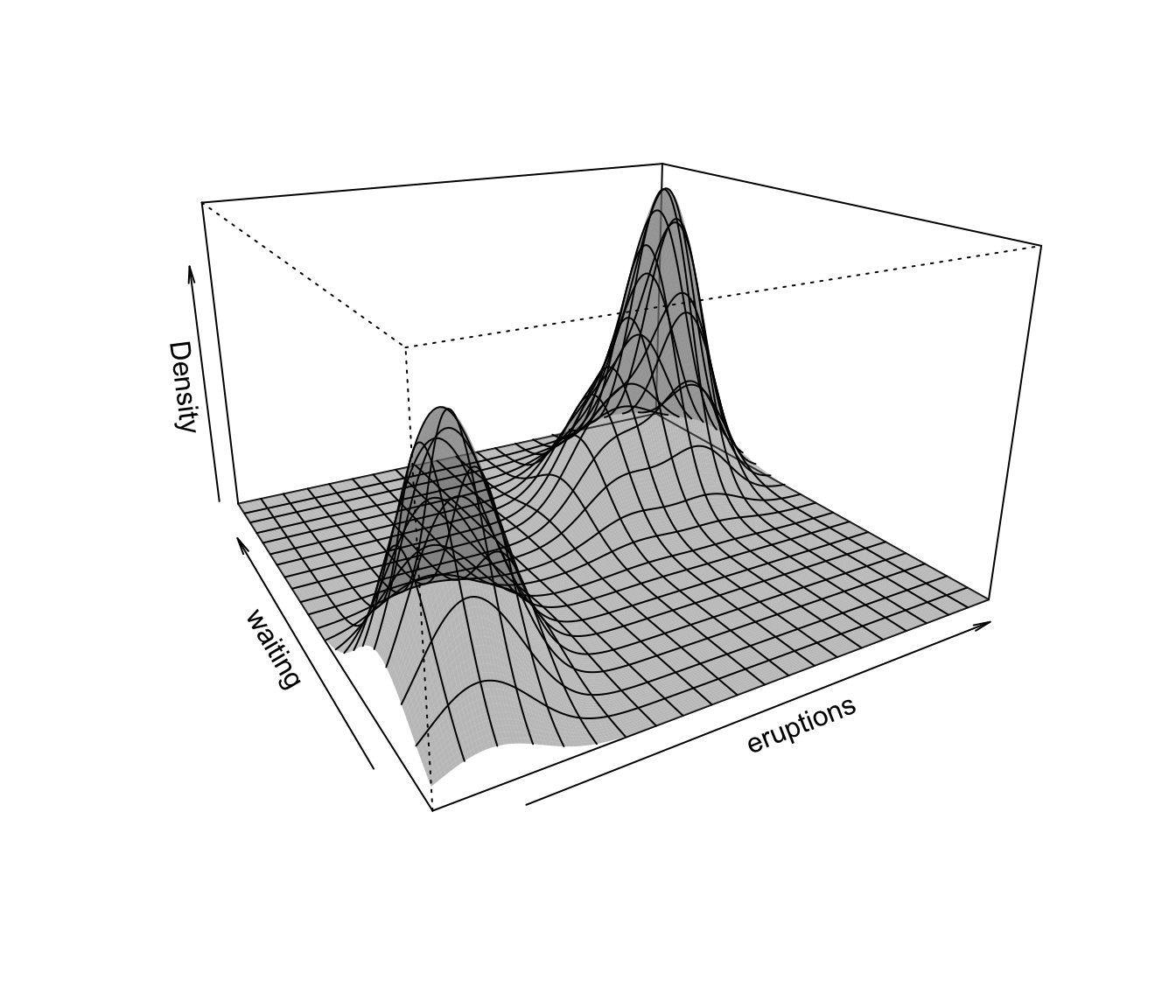plot(dens, what = "density", type = "hdr", data = faithful)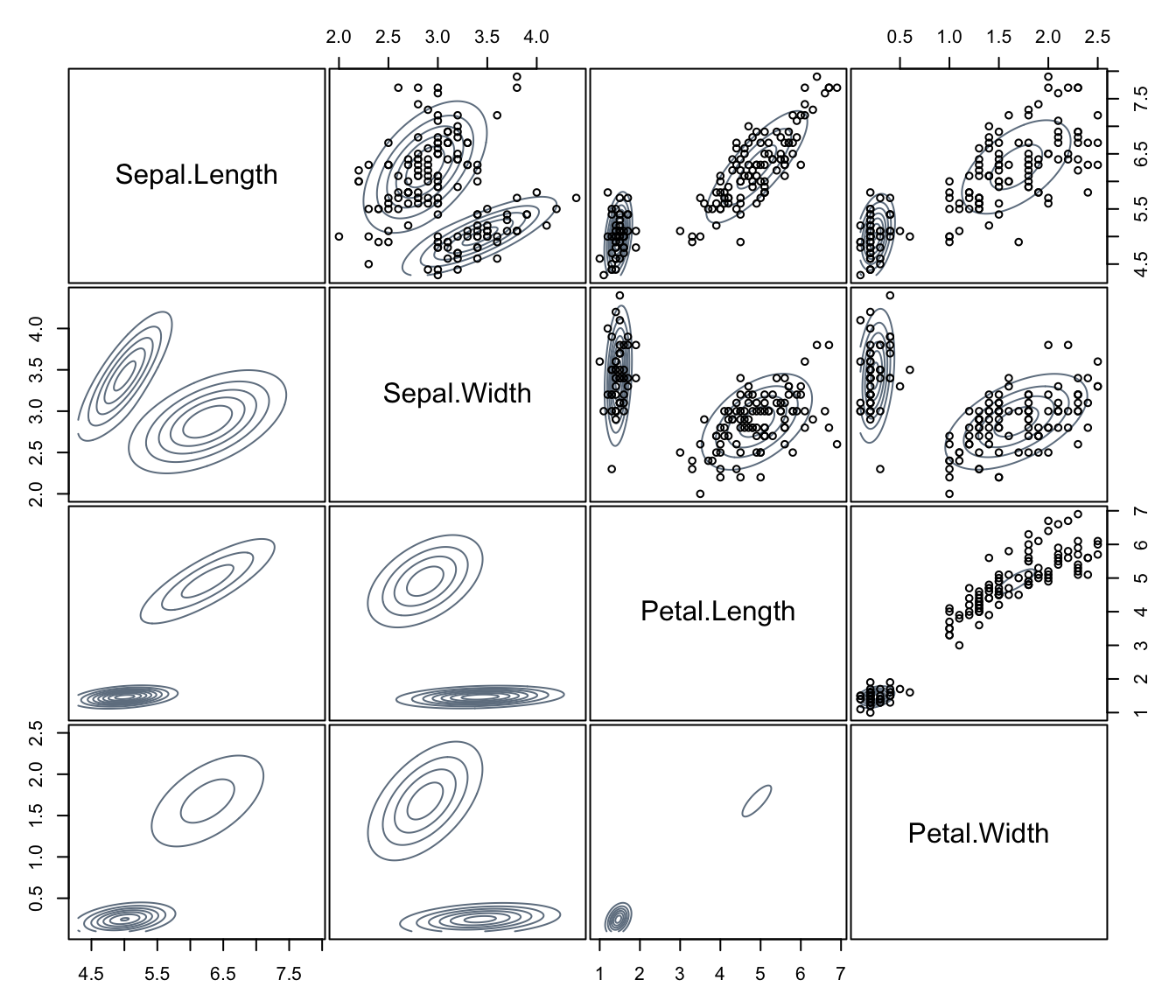plot(dens, what = "density", type = "persp")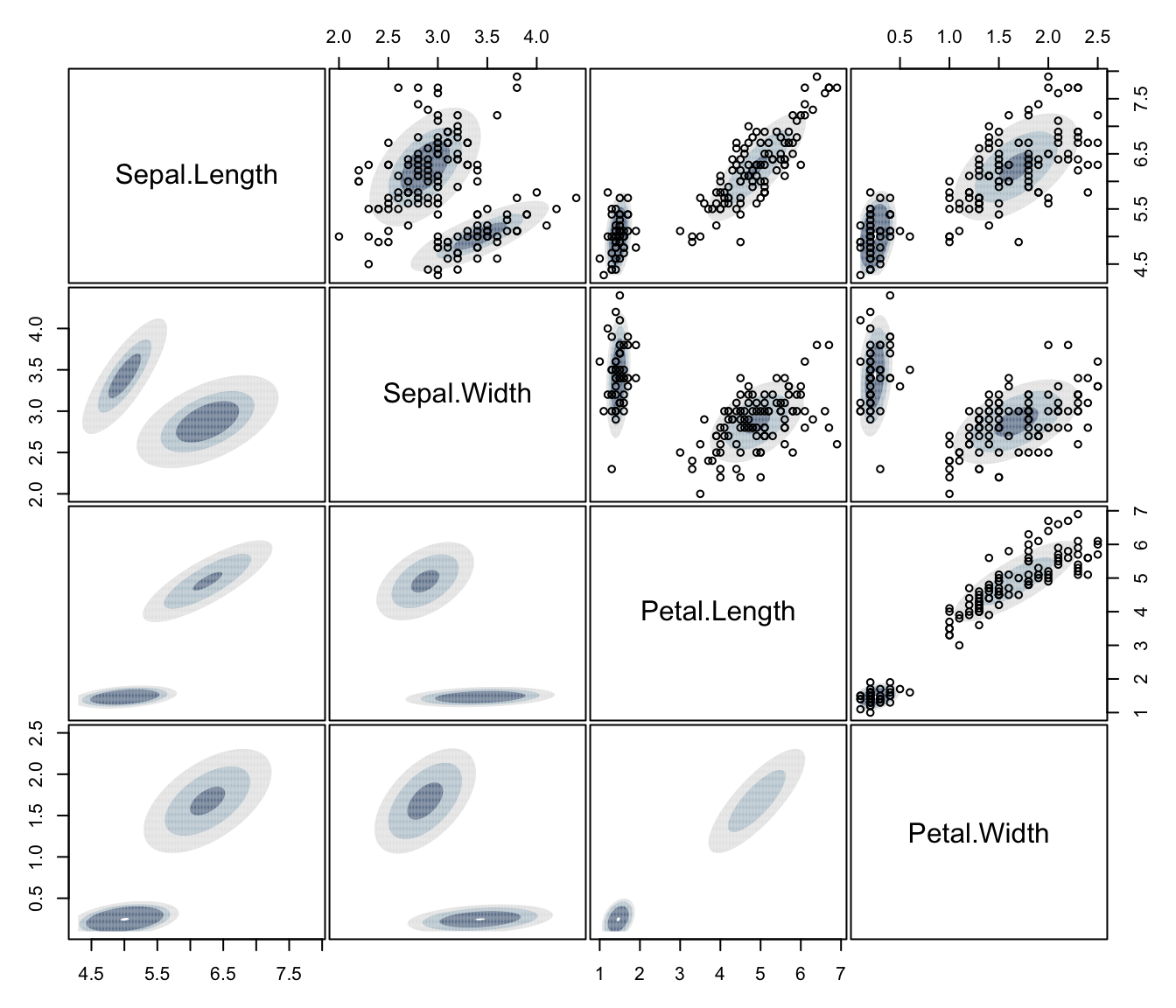dens <- densityMclust(iris[,1:4])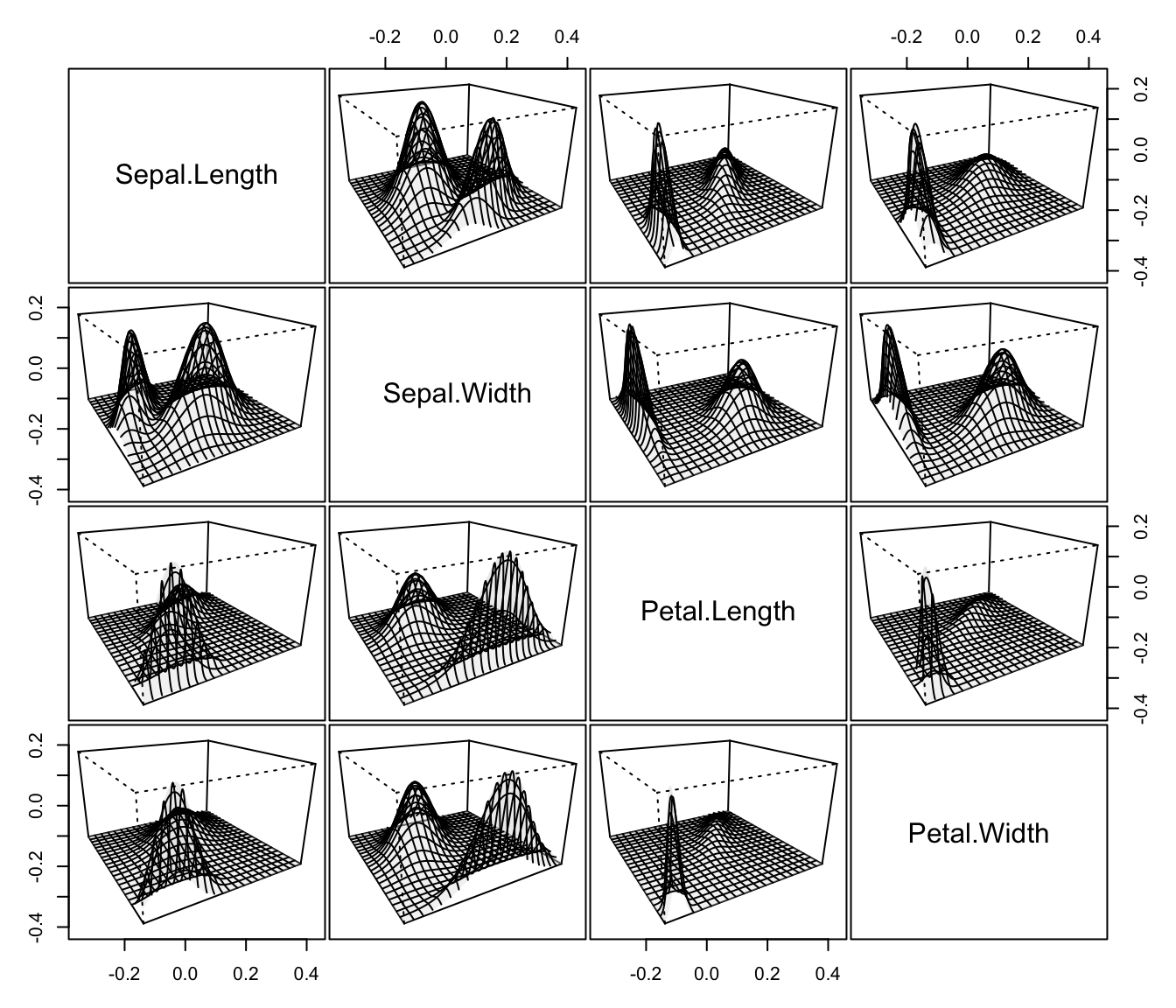summary(dens, parameters = TRUE)
#> -------------------------------------------------------
#> Density estimation via Gaussian finite mixture modeling
#> -------------------------------------------------------
#>
#> Mclust VEV (ellipsoidal, equal shape) model with 2 components:
#>
#>  log-likelihood   n df       BIC       ICL
#>        -215.726 150 26 -561.7285 -561.7289
#>
#> Mixing probabilities:
#>         1         2
#> 0.3333319 0.6666681
#>
#> Means:
#>                   [,1]     [,2]
#> Sepal.Length 5.0060022 6.261996
#> Sepal.Width  3.4280049 2.871999
#> Petal.Length 1.4620007 4.905992
#> Petal.Width  0.2459998 1.675997
#>
#> Variances:
#> [,,1]
#>              Sepal.Length Sepal.Width Petal.Length Petal.Width
#> Sepal.Length   0.15065114  0.13080115   0.02084463  0.01309107
#> Sepal.Width    0.13080115  0.17604529   0.01603245  0.01221458
#> Petal.Length   0.02084463  0.01603245   0.02808260  0.00601568
#> Petal.Width    0.01309107  0.01221458   0.00601568  0.01042365
#> [,,2]
#>              Sepal.Length Sepal.Width Petal.Length Petal.Width
#> Sepal.Length    0.4000438  0.10865444    0.3994018  0.14368256
#> Sepal.Width     0.1086544  0.10928077    0.1238904  0.07284384
#> Petal.Length    0.3994018  0.12389040    0.6109024  0.25738990
#> Petal.Width     0.1436826  0.07284384    0.2573899  0.16808182
plot(dens, what = "density", data = iris[,1:4],
col = "slategrey", drawlabels = FALSE, nlevels = 7)plot(dens, what = "density", type = "hdr", data = iris[,1:4])plot(dens, what = "density", type = "persp", col = grey(0.9))# }